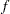FREQ Statement
FREQ variable ;

The variable in the FREQ statement identifies a numeric variable in the data set or one computed through PROC GLIMMIX programming statements that contains the frequency of occurrence for each observation. PROC GLIMMIX treats each observation as if it appearstimes, whereis the value of the FREQ variable for the observation. If it is not an integer, the frequency value is truncated to an integer. If the frequency value is less than 1 or missing, the observation is not used in the analysis. When the FREQ statement is not specified, each observation is assigned a frequency of 1.

The analysis produced by using a FREQ statement reflects the expanded number of observations. For an example of a FREQ statement in a model with random effects, see Example 40.11 in this chapter.